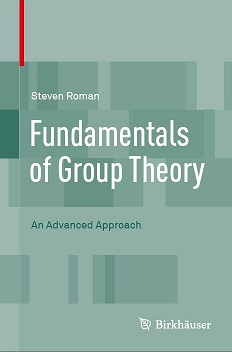# Fundamentals of Group Theory: An Advanced Approach

## Back Copy

Fundamentals of Group Theory provides an advanced look at the basic theory of groups. Standard topics in the field are covered alongside a great deal of unique content. There is an emphasis on universality when discussing the isomorphism theorems, quotient groups and free groups as well as a focus on the role of applying certain operations, such as intersection, lifting and quotient to a "group extension". Certain concepts, such as subnormality, group actions and chain conditions are introduced perhaps a bit earlier than in other texts at this level, in the hopes that the reader would acclimate to these concepts earlier.

Some additional features of the work include:

• An historical look at how Galois viewed groups.
• The problem of whether the commutator subgroup of a group is the same as the set of commutators of the group, including an example of when this is not the case.
• The subnormal join property, that is, the property that the join of two subnormal subgroups is subnormal.
• Cancellation in direct sums.
• A complete proof of the theorem of Baer characterizing nonabelian groups with the property that all of their subgroups are normal.
• A somewhat more in depth discussion of the structure of p-groups, including the nature of conjugates in a p-group, a proof that a p-group with a unique subgroup of any order must be either cyclic (for p>2) or else cyclic or generalized quaternion (for p=2) and the nature of groups of order p^n that have elements of order p^(n-1).
• A discussion of the Sylow subgroups of the symmetric group in terms of wreath products.
• An introduction to the techniques used to characterize finite simple groups.
• Birkhoff's theorem on equational classes and relative freeness.
• This book is suitable for a graduate course in group theory, part of a graduate course in abstract algebra or for independent study. It can also be read by advanced undergraduates. The book assumes no specific background in group theory, but does assume some level of mathematical sophistication on the part of the reader.